# Chemical Equations and Reactions Chemical Reactions l A

• Slides: 37Chemical Equations and Reactions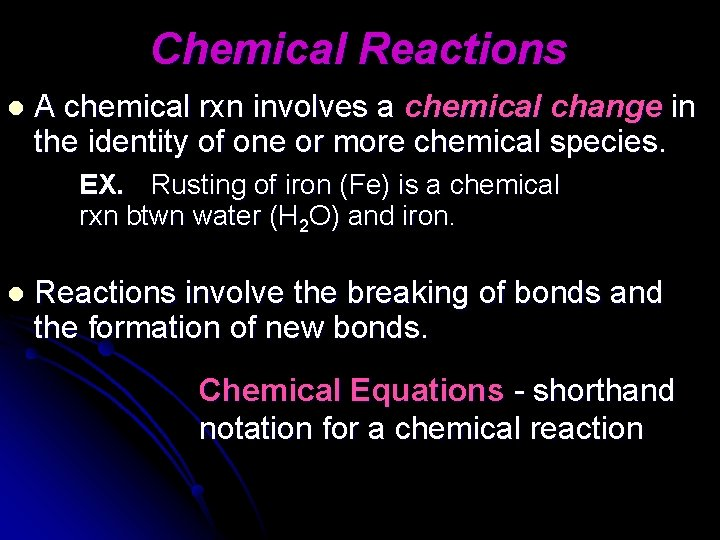Chemical Reactions l A chemical rxn involves a chemical change in the identity of one or more chemical species. EX. Rusting of iron (Fe) is a chemical rxn btwn water (H 2 O) and iron. l Reactions involve the breaking of bonds and the formation of new bonds. Chemical Equations - shorthand notation for a chemical reactionEvidence of Chemical Reactions • Release of a gas. • Formation of a solid (precipitate) in solution. • Heat is produced or absorbed (temp changes) • Color changes. • Light is absorbed or emitted. • Changes in electrical or magnetic properties.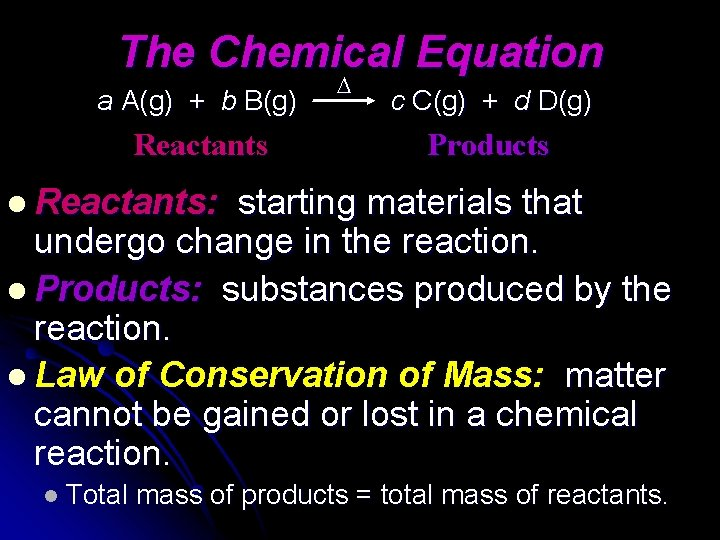The Chemical Equation a A(g) + b B(g) Reactants c C(g) + d D(g) Products l Reactants: starting materials that undergo change in the reaction. l Products: substances produced by the reaction. l Law of Conservation of Mass: matter cannot be gained or lost in a chemical reaction. l Total mass of products = total mass of reactants.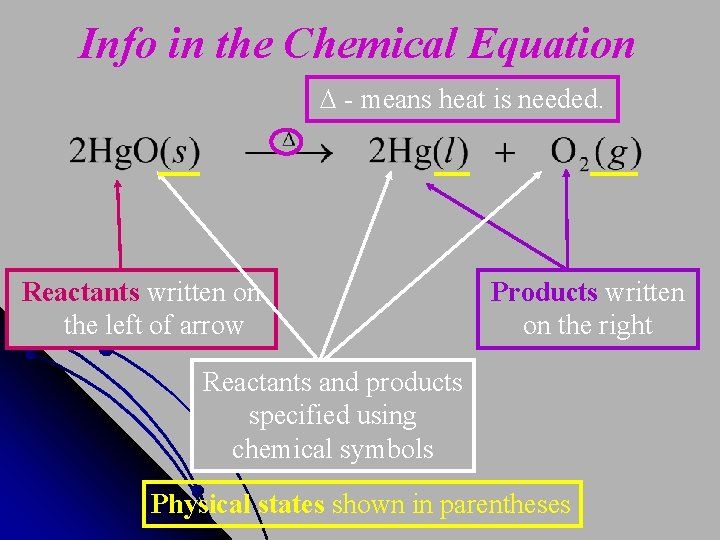Info in the Chemical Equation - means heat is needed. Reactants written on the left of arrow Products written on the right Reactants and products specified using chemical symbols Physical states shown in parenthesesInfo in the Chemical Equation Coefficients: tells how many molecules of each substance are involved in the reaction. • Remember the Conservation of Mass • The equation must be balanced. – All atoms in reactants must also be in products!!Balancing Equations Steps: 1)Must have the same type of elements on each side of the equation. 2)Check for the same # atoms of each element on each side of the equation. 3)Use coefficients in front of each substance to balance the equation.Additional Tips for Balancing NEVER change subscripts, place coefficients in front of the chemical formula l Treat polyatomic ions as a single unit if it is not changed during the rxn l If an element appears in more than one cmpd on the same side of the equation, balance it last l If there is an even # of atoms of an element on one side of the equation & an odd # on the other side, place a 2 in front of the cmpd with the odd # of atoms lMg +2 Na. Cl Mg. Cl 2 + 2 Na Mg Na Cl 1 1 2 x 1 2Balancing Chemical Equations l Consider the following reaction: H 2 (g) + O 2 (g) H 2 O(l) l Is the equation balanced? NO It must be balanced by changing coefficients RIGHT: 2 H 2 (g) + O 2 (g) 2 H 2 O(l) WRONG: H 2 (g) + O 2 (g) H 2 O 2 (l)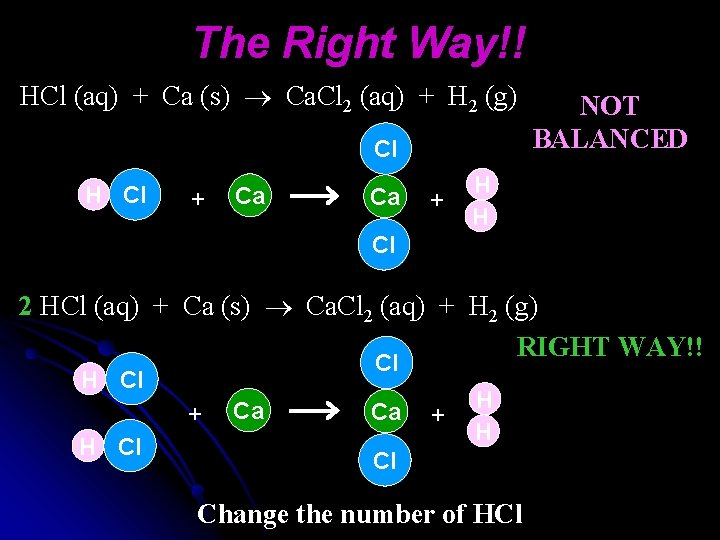The Right Way!! HCl (aq) + Ca (s) Ca. Cl 2 (aq) + H 2 (g) Cl H Cl + Ca Ca + NOT BALANCED H H Cl 2 HCl (aq) + Ca (s) Ca. Cl 2 (aq) + H 2 (g) RIGHT WAY!! Cl H Cl + H Cl Ca Ca + H H Cl Change the number of HCl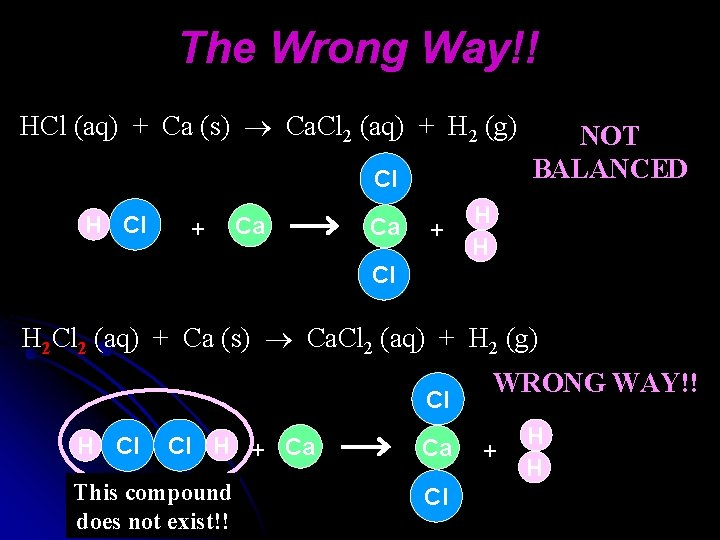The Wrong Way!! HCl (aq) + Ca (s) Ca. Cl 2 (aq) + H 2 (g) Cl H Cl + Ca Ca + NOT BALANCED H H Cl H 2 Cl 2 (aq) + Ca (s) Ca. Cl 2 (aq) + H 2 (g) Cl H Cl Cl H + Ca This compound does not exist!! Ca Cl WRONG WAY!! + H HBalance the following equations: 1. C 2 H 2 + O 2 CO 2 + H 2 O 2. Ag. NO 3 + Fe. Cl 3 Fe(NO 3)3 + Ag. Cl 3. C 2 H 6 + O 2 CO 2 + H 2 O 4. N 2 + H 2 NH 33 types of Rxns involving Energy: Endothermic - thermal energy absorbed during rxn Exothermic - thermal energy given off during rxn Chemiluminescence - light given off during rxn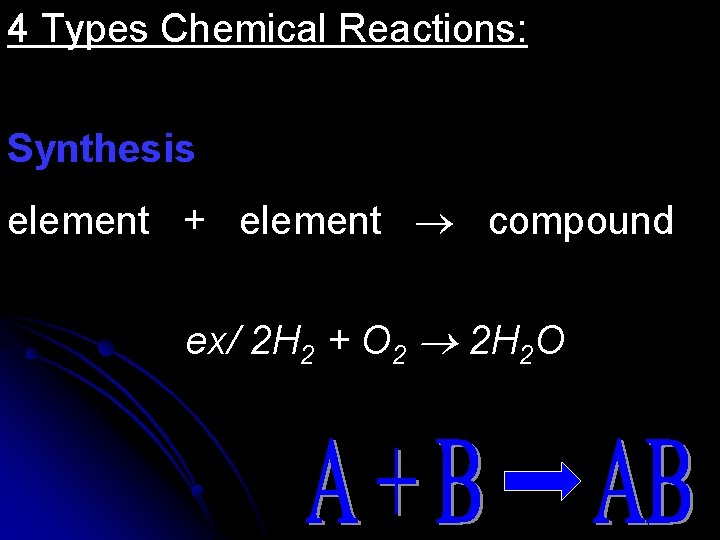4 Types Chemical Reactions: Synthesis element + element compound ex/ 2 H 2 + O 2 2 H 2 O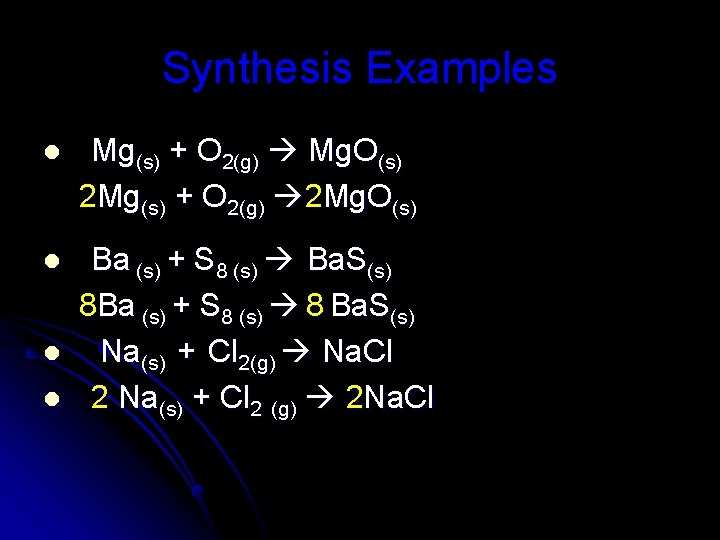Synthesis Examples l Mg(s) + O 2(g) Mg. O(s) 2 Mg(s) + O 2(g) 2 Mg. O(s) l Ba (s) + S 8 (s) Ba. S(s) 8 Ba (s) + S 8 (s) 8 Ba. S(s) Na(s) + Cl 2(g) Na. Cl 2 Na(s) + Cl 2 (g) 2 Na. Cl l lDecomposition compound element + element ex/ 2 H 2 O 2 2 H 2 O + O 2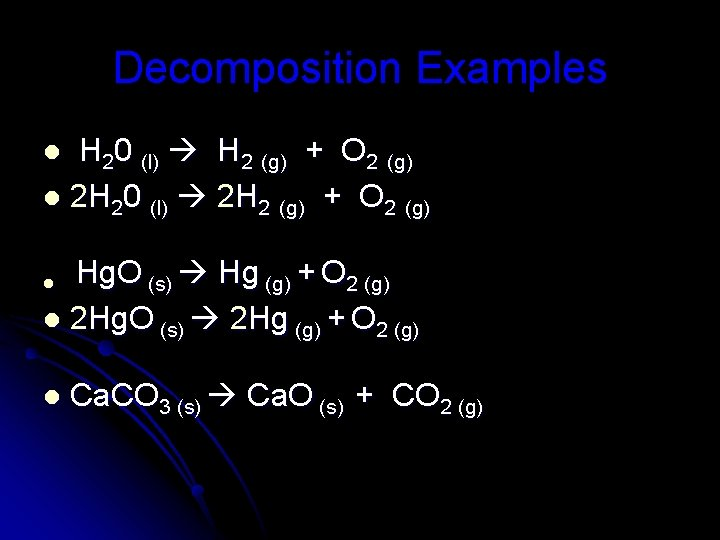Decomposition Examples H 20 (l) H 2 (g) + O 2 (g) l 2 H 20 (l) 2 H 2 (g) + O 2 (g) l Hg. O (s) Hg (g) + O 2 (g) l 2 Hg. O (s) 2 Hg (g) + O 2 (g) l l Ca. CO 3 (s) Ca. O (s) + CO 2 (g)Single Replacement elem + comp replace either the cation or the anion Mg + 2 HCl H 2 + Mg. Cl 2 Ba + Fe. Br 2 Ba. Br 2 + FeSingle Replacement Examples 2 Al(s) + 3 Pb(NO 3)2 (aq) 3 Pb(s) + 2 Al(NO 3)3 (aq) l Al replaces Pb l Cl 2(g) + 2 KBr(aq) 2 KCl(aq) + Br 2(g) l Cl replaces BrDouble Replacement comp + comp ex/ Fe. Cl 3 + Na. OH Na. Cl + Fe(OH)3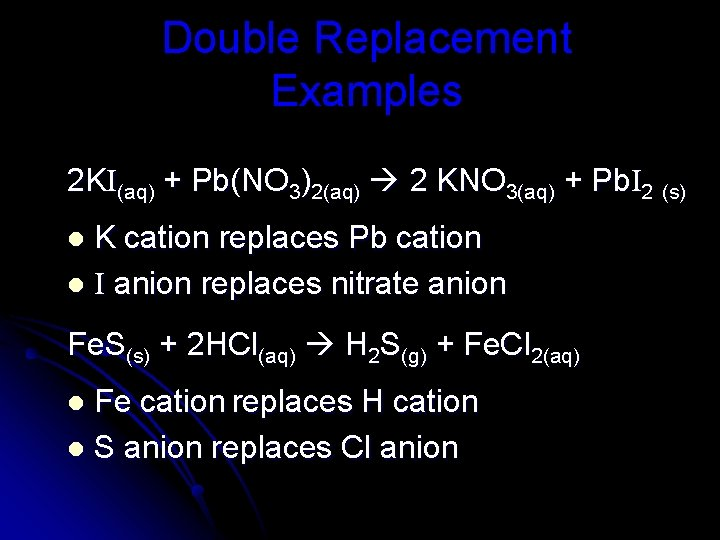Double Replacement Examples 2 KI(aq) + Pb(NO 3)2(aq) 2 KNO 3(aq) + Pb. I 2 (s) K cation replaces Pb cation l I anion replaces nitrate anion l Fe. S(s) + 2 HCl(aq) H 2 S(g) + Fe. Cl 2(aq) Fe cation replaces H cation l S anion replaces Cl anion l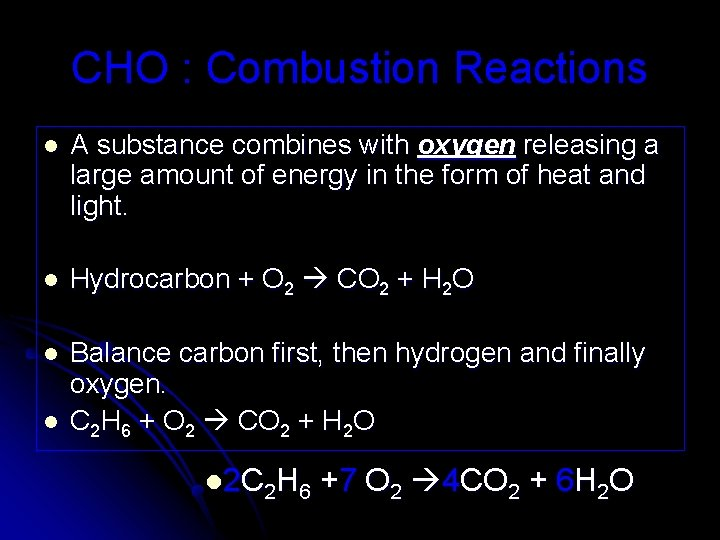CHO : Combustion Reactions l A substance combines with oxygen releasing a large amount of energy in the form of heat and light. l Hydrocarbon + O 2 CO 2 + H 2 O l Balance carbon first, then hydrogen and finally oxygen. C 2 H 6 + O 2 CO 2 + H 2 O l l 2 C 2 H 6 +7 O 2 4 CO 2 + 6 H 2 OCombustion Example: l CH 4 (g) + O 2 (g) CO 2 (g) + H 2 O (g) l CH 4 (g) + 2 O 2 (g) CO 2 (g) + 2 H 2 O (g) l C 3 H 8 (g) + O 2 (g) CO 2 (g) + H 2 O (g) l C 3 H 8 (g) + 5 O 2 (g) 3 CO 2 (g) + 4 H 2 O (g)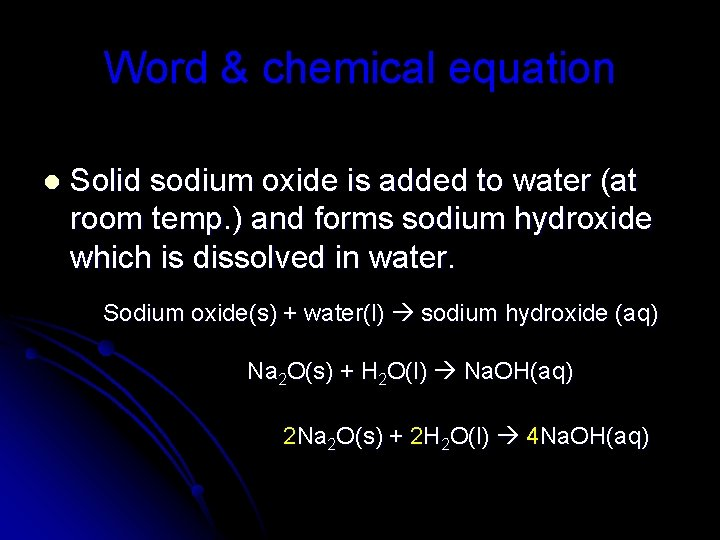Word & chemical equation l Solid sodium oxide is added to water (at room temp. ) and forms sodium hydroxide which is dissolved in water. Sodium oxide(s) + water(l) sodium hydroxide (aq) Na 2 O(s) + H 2 O(l) Na. OH(aq) 2 Na 2 O(s) + 2 H 2 O(l) 4 Na. OH(aq)Word Equations l Write a word equation to describe the reaction of methane as it burns in the air to produce carbon dioxide and water vapor. l. Methane + oxygen carbon dioxide + water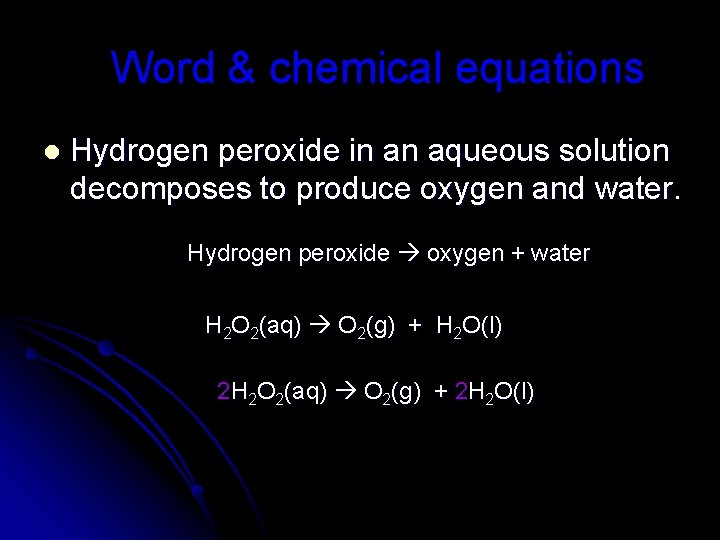Word & chemical equations l Hydrogen peroxide in an aqueous solution decomposes to produce oxygen and water. Hydrogen peroxide oxygen + water H 2 O 2(aq) O 2(g) + H 2 O(l) 2 H 2 O 2(aq) O 2(g) + 2 H 2 O(l)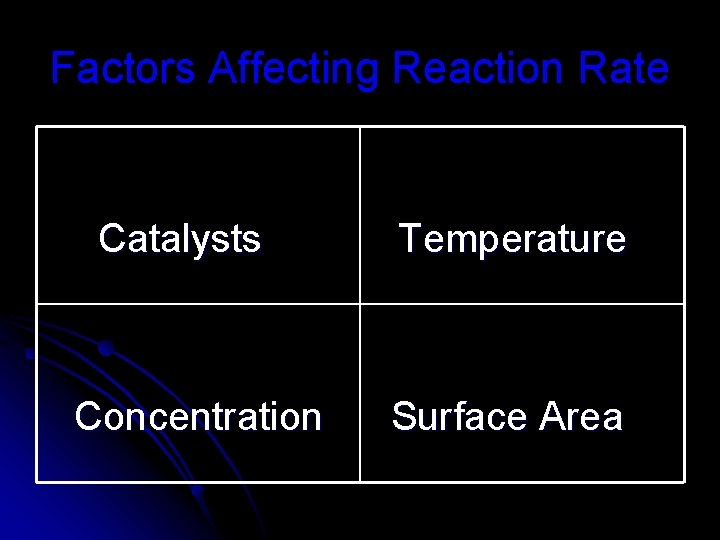Factors Affecting Reaction Rate Catalysts Concentration Temperature Surface AreaCatalysts – speed up chemical rxn but are not used up in the rxn Inhibitors – combine w/ one of the reactants so it can’t react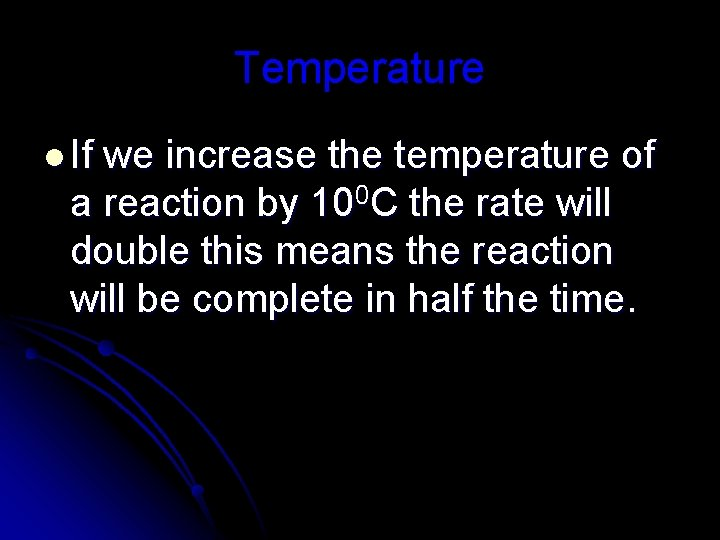Temperature l If we increase the temperature of a reaction by 100 C the rate will double this means the reaction will be complete in half the time.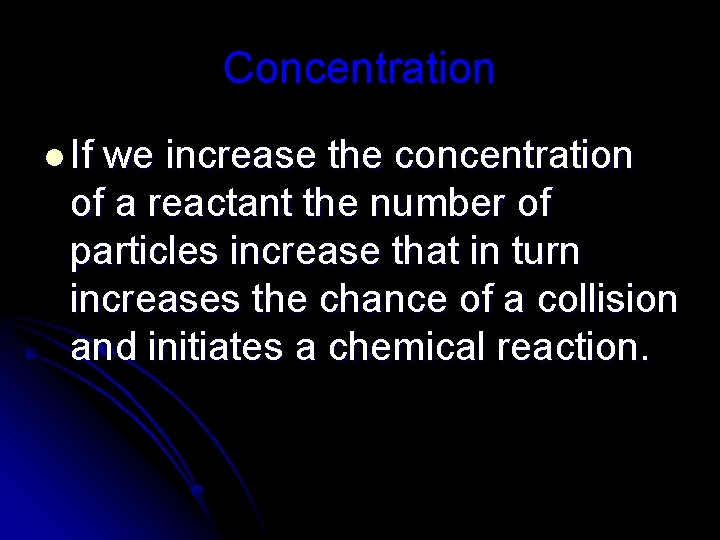Concentration l If we increase the concentration of a reactant the number of particles increase that in turn increases the chance of a collision and initiates a chemical reaction.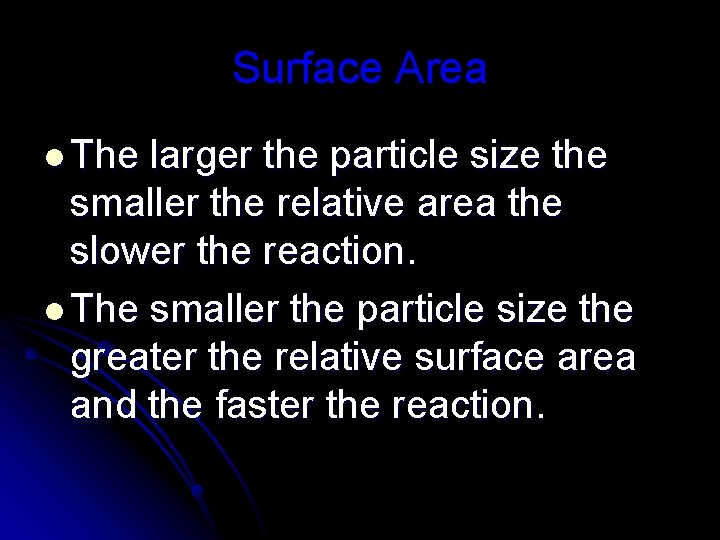Surface Area l The larger the particle size the smaller the relative area the slower the reaction. l The smaller the particle size the greater the relative surface area and the faster the reaction.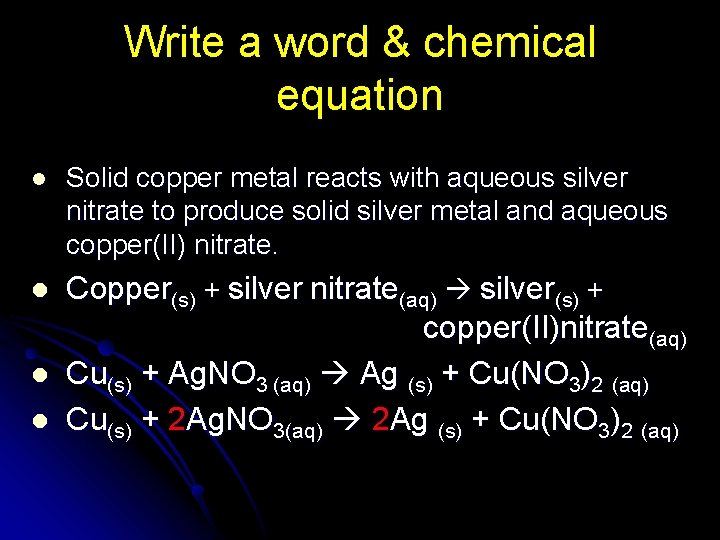Write a word & chemical equation l Solid copper metal reacts with aqueous silver nitrate to produce solid silver metal and aqueous copper(II) nitrate. l Copper(s) + silver nitrate(aq) silver(s) + copper(II)nitrate(aq) Cu(s) + Ag. NO 3 (aq) Ag (s) + Cu(NO 3)2 (aq) Cu(s) + 2 Ag. NO 3(aq) 2 Ag (s) + Cu(NO 3)2 (aq) l l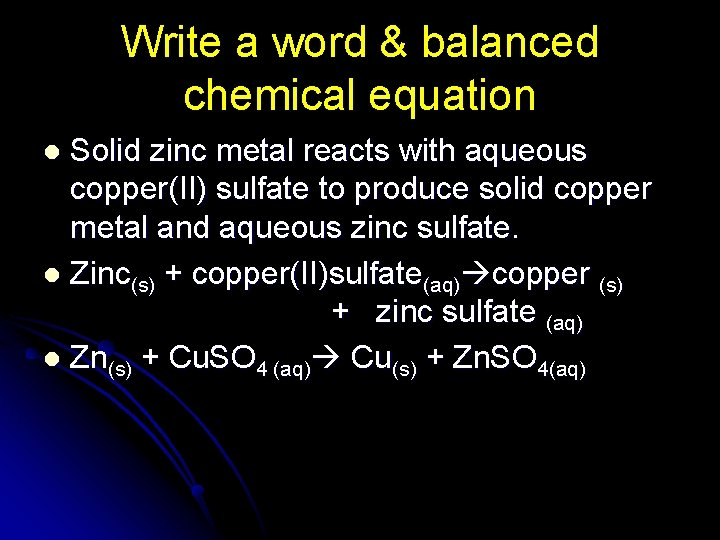Write a word & balanced chemical equation Solid zinc metal reacts with aqueous copper(II) sulfate to produce solid copper metal and aqueous zinc sulfate. l Zinc(s) + copper(II)sulfate(aq) copper (s) + zinc sulfate (aq) l Zn(s) + Cu. SO 4 (aq) Cu(s) + Zn. SO 4(aq) l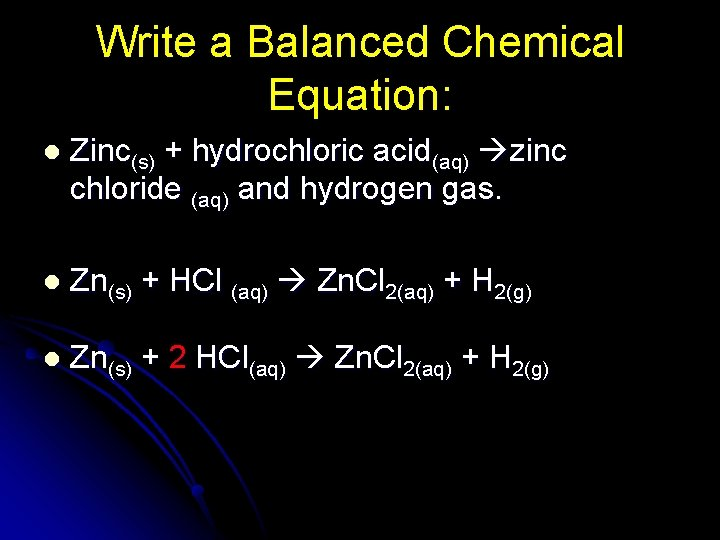Write a Balanced Chemical Equation: l Zinc(s) + hydrochloric acid(aq) zinc chloride (aq) and hydrogen gas. l Zn(s) + HCl (aq) Zn. Cl 2(aq) + H 2(g) l Zn(s) + 2 HCl(aq) Zn. Cl 2(aq) + H 2(g)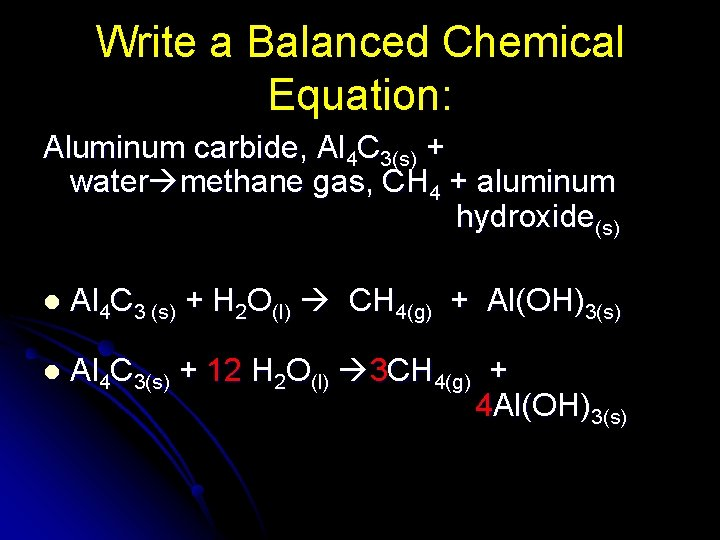Write a Balanced Chemical Equation: Aluminum carbide, Al 4 C 3(s) + water methane gas, CH 4 + aluminum hydroxide(s) l Al 4 C 3 (s) + H 2 O(l) CH 4(g) + Al(OH)3(s) l Al 4 C 3(s) + 12 H 2 O(l) 3 CH 4(g) + 4 Al(OH)3(s)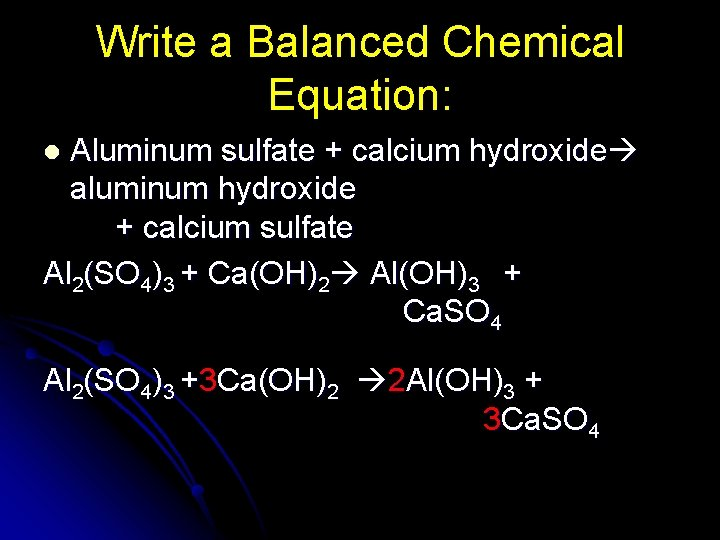Write a Balanced Chemical Equation: Aluminum sulfate + calcium hydroxide aluminum hydroxide + calcium sulfate Al 2(SO 4)3 + Ca(OH)2 Al(OH)3 + Ca. SO 4 l Al 2(SO 4)3 +3 Ca(OH)2 2 Al(OH)3 + 3 Ca. SO 4